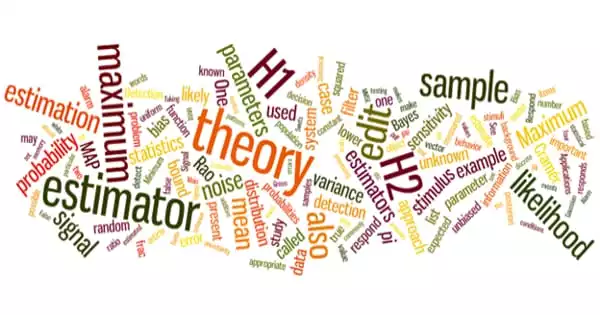# Estimation Theory – a Branch of StatisticsEstimation theory is a branch of statistics concerned with estimating parameter values based on measured empirical data with a random component. It is a crucial mathematical concept that is used in a wide range of communication and signal processing applications. The parameters describe an underlying physical setting in such a way that the value of the parameters affects the distribution of the measured data. This theory is useful in estimating the desired information in received data and is thus used in a wide range of applications ranging from radar to speech processing.

Estimation theory is a branch of statistics and signals processing concerned with estimating parameter values based on measured/empirical data with a random component. The parameters describe an underlying physical setting in such a way that the value of the parameters affects the distribution of the measured data. Using the measurements, an estimator attempts to approximate the unknown parameters. Read In estimation theory, two approaches are commonly used.

• The probabilistic approach assumes that the measured data is random with probability distribution dependent on the parameters of interest
• The set-membership approach assumes that the measured data vector belongs to a set which depends on the parameter vector.

Estimation process

The entire goal of estimation theory is to arrive at an estimator, preferably one that can be implemented and used. The estimator uses the measured data as input and generates a parameter estimate.

It is also preferable to obtain an estimator that is optimal. Estimator optimality is typically defined as achieving the lowest average error across a class of estimators, such as a minimum variance unbiased estimator. The class is the set of unbiased estimators in this case, and the average error measure is variance (average squared error between the value of the estimate and the parameter). However, optimal estimators are not always available.

Examples

For example, it is desirable to estimate the proportion of a voter population who will vote for a specific candidate. The parameter sought is that proportion; the estimate is based on a small random sample of voters. Alternatively, it is desired to estimate the likelihood of a voter voting for a specific candidate based on demographic characteristics such as age.

In radar, for example, the goal is to determine the range of objects (airplanes, boats, etc.) by analyzing the two-way transit timing of received echoes of transmitted pulses. Because the reflected pulses are unavoidably embedded in electrical noise, their measured values are randomly distributed, necessitating an estimate of the transit time.

In electrical communication theory, for example, measurements that contain information about the parameters of interest are frequently associated with a noisy signal.

In estimation theory, the measured data is assumed to be random, with a probability distribution dependent on the parameters of interest. In electrical communication theory, for example, measurements containing information about the parameters of interest are frequently associated with a noisy signal. The problem would be deterministic if there was no randomness or noise, and estimation would not be required.